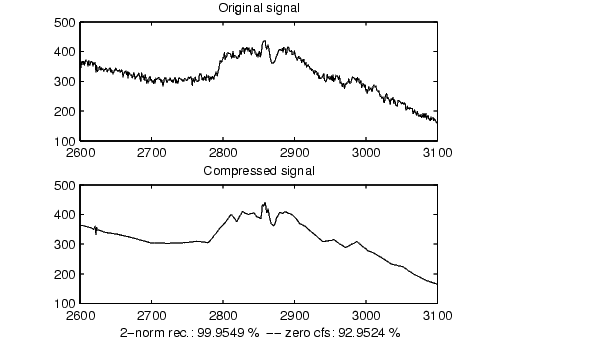Wavelet Toolboxwdcbm

Thresholds for wavelet 1-D using Birge-Massart strategy

Syntax

• ```[THR,NKEEP] = wdcbm(C,L,ALPHA)
[THR,NKEEP] = wdcbm(C,L,ALPHA,M)
```

Description

[THR,NKEEP] = wdcbm(C,L,ALPHA,M) returns level-dependent thresholds THR and numbers of coefficients to be kept NKEEP, for de-noising or compression. THR is obtained using a wavelet coefficients selection rule based on the Birge-Massart strategy.

[C,L] is the wavelet decomposition structure of the signal to be de-noised or compressed, at level j` = length(L)-2`.
ALPHA and M must be real numbers greater than 1.

THR is a vector of length j, THR(i) contains the threshold for level i.

NKEEP is a vector of length j, NKEEP(i) contains the number of coefficients to be kept at level i.

j, M and ALPHA define the strategy:

• At level j+1 (and coarser levels), everything is kept.
• For level i from 1 to j, the ni largest coefficients are kept with ni = M  (j+2-i)ALPHA.

Typically ALPHA = 1.5 for compression and ALPHA = 3 for de-noising.

A default value for M is M = L(1), the number of the coarsest approximation coefficients, since the previous formula leads for i = j+1, to nj+1 = M = L(1). Recommended values for M are from L(1) to 2*L(1).

wdcbm(C,L,ALPHA) is equivalent to wdcbm(C,L,ALPHA,L(1)).

Examples

• ```% Load electrical signal and select a part of it.
x = leleccum(indx);

% Perform a wavelet decomposition of the signal
% at level 5 using db3.
wname = 'db3'; lev = 5;
[c,l] = wavedec(x,lev,wname);

% Use wdcbm for selecting level dependent thresholds
% for signal compression using the adviced parameters.
alpha = 1.5; m = l(1);
[thr,nkeep] = wdcbm(c,l,alpha,m)

thr =
19.5569   17.1415   20.2599   42.8959   15.0049

nkeep =
1     2     3     4     7

% Use wdencmp for compressing the signal using the above
% thresholds with hard thresholding.
[xd,cxd,lxd,perf0,perfl2] = ...
wdencmp('lvd',c,l,wname,lev,thr,'h');

% Plot original and compressed signals.
subplot(211), plot(indx,x), title('Original signal');
subplot(212), plot(indx,xd), title('Compressed signal');
xlab1 = ['2-norm rec.: ',num2str(perfl2)];
xlab2 = [' %  -- zero cfs: ',num2str(perf0), ' %'];
xlabel([xlab1 xlab2]);```

```wden, wdencmp, wpdencmp ```wcodemat wdcbm2